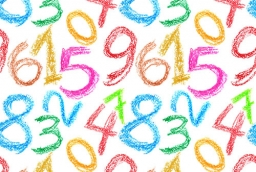# Probability 42081

What is the probability of any two-digit natural number
a) is divisible by seven,
b) is divisible by nine,
c) is not divisible by five.

p1 =  0.1444
p2 =  0.1111
p3 =  0.8

### Step-by-step explanation:Did you find an error or inaccuracy? Feel free to write us. Thank you!

Tips for related online calculators
Would you like to compute the count of combinations?